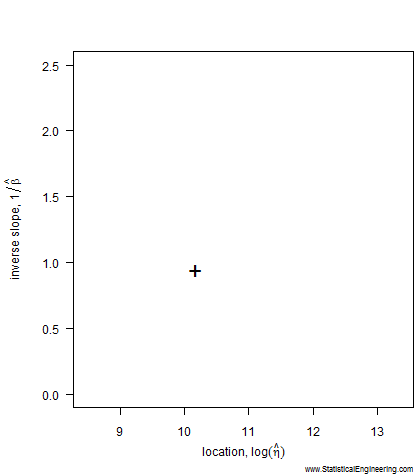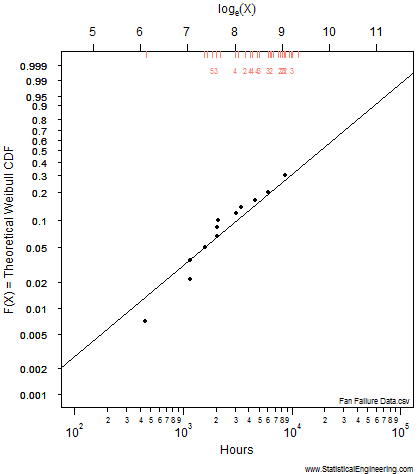## Censored Likelihood

### Likelihood is “the probability of the data.”

#### It is proportional to the probability that the experiment turned out the way it did.

So some statistical model parameters are more likely than others because they explain the observed failures better than other values. We choose the “best” parameter values, i.e. those that maximize the likelihood, which are called, not surprisingly, “maximum likelihood parameter estimates.”  We’ll use the Weibull as an example.

The most likely parameter values, given the data, for the Weibull slope, $$\beta$$, and location, $$\eta$$, are the “$$+$$” in Figure 1, but other values are also plausible, although less likely. That ($$\beta, \eta$$) pair produces the best fit of the data, shown as the black line in Figure 2.

The dataset below is severely right-censored: 58 of the 70 components were removed from service at various times before they failed, and are indicated by red tic-marks along the top of figure 2. 12 failed, plotted as points. The data are from Meeker and Escobar (1998), Statistical Methods for Reliability Data, Wiley, Table C-1, p630.The most likely parameter values, given the data, for the Weibull slope, $$\beta$$, and location, $$\eta$$, are the “$$+$$” but other values are also plausible, although less likely. That ($$\beta$$, $$\eta$$) pair produces the best fit of the data, shown as the black line in Figure 2.